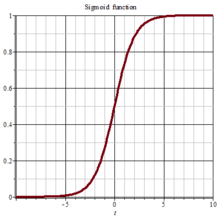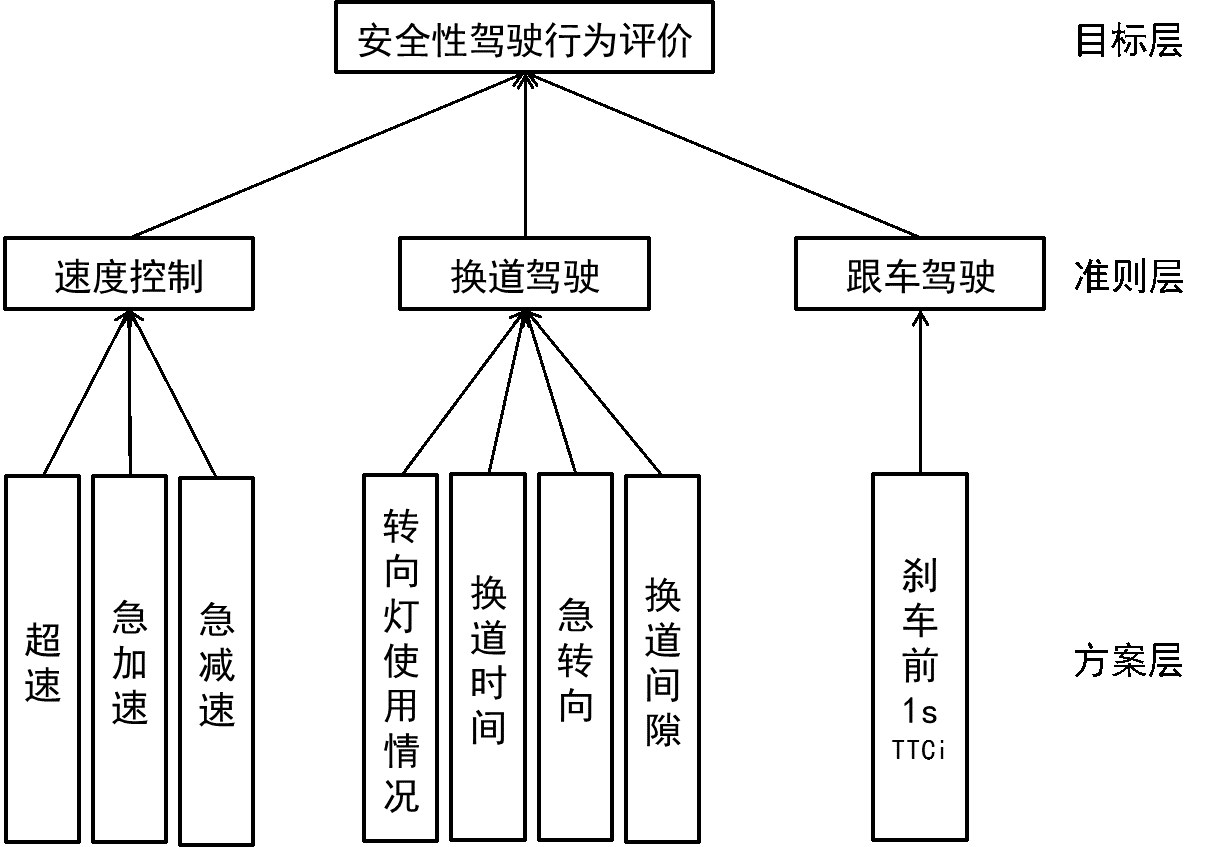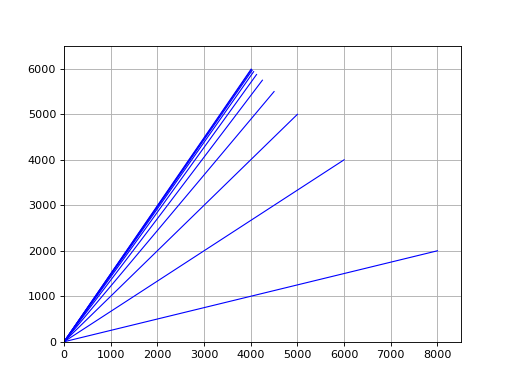@devilogic 2017-12-31T11:37:56.000000Z 字数 19285 阅读 1126

# 玩命的销售日志 2017.12.31

日志$\quad$前段时间刚从大武汉吃热干面回来，连续十几天的出差，感觉人快废掉了。最近嗓子发炎加上天气变冷又感冒了。第一次觉得自己身体抗不住了。话说武汉正宗的热干面刷新了我对热干面的认识，在北京上地某XX鸟饭店吃的就是坨屎。
$\quad$干了半年销售和售前。唯一的心得体会就是累，是真累，还特消耗时间。除了看小说的时间增多了，其余的时间都少了。原来做技术的时候，感觉做销售有什么啊！不就是每天见见人，聊聊天，出去喝喝酒嘛！有什么啊~~~。干过销售以后，我收回以前所说的屁话。唯一感想就是写程序是闹心，做销售是恶心
$\quad$前几天我从小长耍到大的哥们千千说想和我一起跑跑IT类销售，看看是否能帮我在我老家少得可怜的科技企业中推销一些我们的产品。我答应了，就一起跑了趟上海。
$\quad$话说千千是一个在银行待了10年的老行员了，最初开始给银行推销信用卡，随后又做消费贷、车贷与房贷。据说当初推销信用卡的时候直接推开某些政企单位领导办公室的门，死皮赖脸的要人家全单位办信用卡。貌似他第一辆车就是这样来的。
$\quad$今年年初从他一个搞设计的朋友那边得到灵感，想在网上卖香薰蜡烛。我当初还以为丫是说笑。一个大老爷们搞这种小资情调的玩意。但是这货从有想法到设计自己的品牌再到建立网店外加建立渠道一共也就两周时间。人们都说一流的行动力比一流的Idea要重要的多。难得的是千千既有行动力又有Idea。从确定走高逼格小众香薰路线到整套的品牌VI设计一周时间，随后自己在家熬香薰，结果工艺不行。被逼无奈丫开始联系代理商一共又花了一周。运营了一个月小店就盈利了。丫又找来我另外一个哥们四强性欲强，性欲强，性欲强，性欲强，重要的事情说四遍两人合伙搞这家小店，四强原本也是安全圈的程序员，搞了几年安全觉得赚钱太少，就去开了一个taobao店卖做蛋糕的模具，做了一年多在成都买了两套房，三辆车（宝马，奥迪，霸道）。当初写程序的时候真没看出他这么会做生意。• 脸是什么玩意？
• 一定要包装好看

• 认清自己的客户群体 - 小资情调生活美学装逼文艺女青年
• 锁定自己的产品方向 - 主要经营小众品牌香薰，少量周边产品

$\quad$最近出差确实有点多，没时间研究什么技术。也就在飞机与高铁上随便写两行代码练练手。吃饭的手艺不能丢

$\quad$在旅途中看小说，写代码之余也思考下我们这个行业。这个行业内的几家企业真是一切跟的风口走。风往哪里刮，牛就往哪里吹。当物联网很火的时候，保护两个电饭锅，空调的APP就叫涉及物联网安全。车联网起风了，那么去给车厂做做APP 保护就叫车联网布局。貌似某家公司还研究过区块链安全。总之哪个行业火，就去给哪个行业做APP加固，就说在涉及这个行业，是为这个行业定制的安全加固方案。其实都很扯蛋。还有些产品也是纯粹概念，反正安全产品对于用户来说，太难进行验证。
$\quad$梆梆有个产品叫做白盒加密，还为此写了两篇哄小孩子的文章。其实这里想说一下，白盒加密是一个概念，不是一个特定的技术或者算法，更不能用来定义一个产品。这个概念就是指算法与密钥在暴露的情况下，如何保证加密的安全。其实软件保护本身就是白盒加密。不过很少有客户明白。我们就是太缺少这方面的经验，无论是产品概念还是文档形式。

$\quad$我发现一点是企业对于包装这个事情是给两方面看的，一是老实的客户，二是自以为是的VC。前者是对自己的产品进行包装来骗客户，后者是对自己的公司战略进行包装来骗融资。

$\quad$想想我们确实应该和千千去学习一下，在网上卖带香味的蜡烛和用玻璃瓶装的火柴，就说自己从事生活美学方面的工作。并且对此深信不疑，为此还专门去了日本，说是要学习体验。

$\quad$不过对于准备一辈子都要做程序员的人来说还是聊聊技术问题吧！都说有两样东西每个男人都会喜欢，一个是，一个是。我对车没太多的执念，但对于后者如果在法律允许下我可能会在家里搞一个军火库出来。但这几天的研究对象是前者，起因是最近正好在看车，其次是因为车联网这么火，就顺手看一下。反正飞机上也没其他的事情可做。
$\quad$正好前段时间有朋友给了我篇关于驾驶安全方面的论文，我也从网上搜了一些。想自己闲暇无事实现一套这样的程序。从驾驶风格来给安全性和经济性打个分。所以就有了以下的文章。

## 问题分析

$\quad$下面主要阐述了驾驶安全性评估方面的算法。我的想法是这样的，程序的输入是一组一辆车在一段路的驾驶参数，程序对他这段路的驾驶安全性进行打分。以下这套模型是取自一篇清华大学和千方集团的论文。

### 刹车前1s的$TTCi^1$

$\quad TTCi$是描述前后车辆关系的一个物理参数，常用来评价跟车过程中的前后车辆间的危险程度。其计算公式如下：

## 设计算法

$\quad$我们的最终目的是为了求得在一辆车在某一段路的安全行驶分数。这个分数反映了驾驶人在这段路的是否涉及危险驾驶。
$\quad$通过以上的问题分析，准备设计一段程序，通过输入一组长度为$8$的向量$[a_1,a_2,a_3,\ldots,a_8]$。输出得到一个$[0,100]$的整型数，数字越高表示越安全，数字越低表示越不安全。
$\quad$首先我们通过分组将速度控制换道驾驶跟车驾驶。分为三组采取不同的权重进行影响。$[a_1,a_2,a_3],[b_1,b_2,b_3,b_4],[c_1]$三组。分别对应了$[w_{a1},w_{a2},w_{a3}],[w_{b1},w_{b2},w_{b3},w_{b4}],[w_{c1}]$。由于最后一组只有一项所以$w_{c1} = 1$。其中

$\quad$也可以通过一组$8$长度向量通过神经网络直接得到最后的分值。无论哪种方式我们都要得到一组权重，这有两种方式得到，一种是主观的方式，采用AHP算法。一种是客观的方式，通过大量的样本进行训练。因为我们并没有大量的样本，所以我们通过AHP算法进行权重的生成。
$\quad$在此之前我们通过以上有些是布尔，有些是整型,有些是浮点数。我们的系统输入需要做些统一度量的工作，避免输入数值相差过大对输出造成影响。通常状况有以下这样几种 归一化方法：

### 2.平均数方差法

1. 对表中每项进行阀值的规定，当超过一个阀值时，统计次数。
2. 使用最大最小法对每项进行处理使得每个值都在$[0,1]$再使用$sigmod$对其进行压缩使得数值相差太大。

$sigmod$函数将输入挤压到$[0, 1]$区间。$sigmod(x) = \frac{1}{1+e^{-x}}$### 使用次数作计算

1. 间隔$1$秒取一次当前车况状态。通过当前的状态计算出表中的数据。
2. 因为分析到在实际路况中，偶然的违反以上的限定有时候是难免的。所以统计这段路以上过界的次数。以次数来反映整条路段的行驶状况，次数越少得分当然也就越高。
3. 规定一个距离$s$当到达这个距离时计算这个危险分数的分值。
4. 可以在汽车停止后，平均所有$n$个路段的分数，得出最后的分值。
5. 由于车速这个值在不同天气状况以及不同路段是有不同的标准，所以需要汽车传感器在不同的情况进行统计。
6. 由于交规中没有对一些数值进行阀值的规定，例如：超车间距，以及超车时间等。所以在实际系统中还需要涉及这些具体值的限定。

### 直接使用数值作计算

1. 间隔$1$秒取一次当前车况状态，通过当前的车况，只需要将数值传递给网络中进行运算，直接得出此路段分数。
2. 由于这种直接取数值的计算，是当前$1$秒的分数，所以这里可以分别间隔$n$秒后取一次或者连续取$m$秒并做均值。
3. 在汽车停止后，平均所有$n$个路段的分数，得出最后的分值。
4. 由于车速这个值在不同天气状况以及不同路段是有不同的标准，所以需要汽车传感器在不同的情况进行统计。

• 车速，急加速，急减速 数值越大，越危险。分数就越低
• 是否使用换道时间越短、急转向数值越大、换道间隙越小 越危险。分数就越低
• 刹车前1s的$TTCi$越小，越危险。分数就越低

$\quad$这两种方案，都有自己的好处，第一个实现比较简单，需要一些对于抓取的车况数据先进行预先处理。再进行运算。第二种不需要预运算，但是需要设计一个复杂的网络。这里我们使用第二种方案。原因没有实用角度，只是不喜欢预处理数据这部分。
$\quad$这里再次说明一下。如果你有足够的样本 - 使用神经网络进行训练。这需要划分一下目标。因为是百分制而驾驶行为也是连续的，所以需要首先做下空间划分。以达到良好的映射关系。但是总觉得少了点什么。
$\quad$如果没有样本，那么我的想法是使用$AHP$算法进行人为的权重设定。也就是机器学习中所说的先验知识。依靠专家的知识进行权重的设定。

### 2012年中国高速公路事故信息 <- 我们所要依赖的专家知识## 网络设计

1. 归一化处理
2. 环境因素的影响，雪天，晴天对路况的影响
3. 最终得出的分值(百分制)

$inputs = [a_1,a_2,a_3,b_1,b_2,b_3,b_4,c_1,x,y]^T$ 这就构成了我们的输入。

### 预处理层

$\quad$这里并不对$x,y$进行任何处理。应为输入总共有$10$个元素，其中设预处理函数为

#### $x,y$数值分析

$\quad x$其实是一个分段阀值。我们姑且想想汽车可以通过GPS信息结合地图信息获取当前道路情况并传递给我们。那么这里应该传递是当前路段的最高时速$[120,80,70,60,40]$。这里因为是车速所以也直接同样做归一化处理,$y$是能见度，当大于$15$后，将没有任何限制，所以是一个取值$[1,15]$的数值。这里做一下归一化处理直接除以$15$得到一个$[0,1]$之间的数。这里多一个函数专门来处理$y$

### 评分层

$\quad$这个层汇集了三个子网络的输出$[a,b,c]$，通过AHP产生这三项相对重要程度的权重$[w_1,w_2,w_3]$，最终得到一个输出$output$，这个值应该是一个在$[0,1]$之间的数。简单的使用$100$来乘。得到的分数越高，则表示驾驶越安全。
$\quad$接下来就是探讨如何生成权重的事情了。因为是主观确定，所以采用了AHP。下面简单探讨了AHP算法。

## 层次分析法(AHP)

$\quad$首先说明的是以上的评测模型都是建立在一个叫做层次分析法的基础上的。简单的介绍下层次分析法

$\quad$层次分析法是是一种定性分析和定量分析相结合的方法。它通过分析复杂问题所包含的因素及其相互关系,将问题分解为不同的因素,并将这些问题归并成不同的层次,从而形成多层结构。在每一层次,按照一定准则对该层元素进行逐对比较,并按标度定量化
,形成判断矩阵。通过计算判断矩阵的最大特征值以及相对应的正交化特征向量,得出该元素对该准则的权重。在此基础上,可以计算出各层次元素对于该准则的比重。其具体实施步骤如下:

### 1 判断矩阵的构造

$\quad$假设某个物体$A$$n$个部分：$a_1,a_2,\cdots,a_n$，且$a_i \gt 0$，则$a_i / a_j$表示第$i$个部分相对整体$A$而言比第$j$部分重要的倍数，用$a_{ij}$表示，则可以得到如下矩阵：

1. $a_{ij} \gt 0$
2. $a_{ij} = \frac{1}{a_{ji}}$
3. $a_{ii} = 1 (i = 1,2,\cdots,n)$

1. $rank(A) = 1$，且存在唯一的非零特征值$\lambda_{max} = n$，其规范化特征向量$W = (W_1,W_2,W_3,\cdots,W_n$叫做权重向量；
2. $a_{ij} = a_{ik} \cdot a_{kj}(i,j,k=1,2,\cdots,n)$
3. $A$的列向量之和经规范化后的向量就是权重向量；
4. $A$的任一列向量经规范化后的向量就是权重向量；
5. $A$的全部列向量先求每一分量的几何平均，再规范化后的向量就是权重向量

#### 指数标度的标度值和含义：

$a_i$$a_j$同等重要 $e^{0/4}$
$a_i$$a_j$稍微重要 $e^{2/4}$
$a_i$$a_j$相当重要 $e^{4/4}$
$a_i$$a_j$强烈重要 $e^{6/4}$
$a_i$$a_j$极端重要 $e^{8/4}$
$a_i$$a_j$的重要性在上述描述之间 $e^{1/4},e^{3/4},e^{5/4},e^{7/4}$
$a_i$$a_j$不重要的描述 相应标度的倒数

### 2 判断矩阵的一致性判别

1. $A$的每一列向量归一化得到$w_{ij} = a_{ij} / \sum^n_{i=1}a_{ij}$
2. $w_{ij}$按行求和得到$w_{i}=\sum^n_{i=1}w_{ij}$
3. $w_i$归一化得到$W_i = w_i/\sum^n_{i=1}w_i,W = (W_1,W_2,W_3,\cdots,W_n)$即为近似特征向量
4. 计算$\lambda = \sum^n_{i=1}\frac{AW^T}{nW_i}$，将其作为最大特征根的近似值。

### 3 专家信息的预处理

$\quad$定理1知，当$A$为一致性矩阵时，$a_{ij} = a_{ik} \cdot a_{kj}$，因此用$a_{ij}$$a_{ik} \cdot a_{kj}$的偏差来检测判断矩阵的一致性是有效的。
$\quad$用矩阵的最大偏差值$s$和均方差$\sigma$来检验矩阵的一致性，最大偏差值$s$和均方差$\sigma$的定义如下：

$s$$\sigma$的值越小，说明矩阵的一致性越好。

### 特征值与特征向量

$\quad$为求得$1$年后结婚女性和单身女性的人数，我们将向量$w_0 = (8000, 2000)^T$乘以

$1$年后结婚女性和单身女性的人数为

import numpy as npfrom matplotlib.lines import Line2Dimport matplotlib.pyplot as pltfigure, ax = plt.subplots()ax.set_xlim(left=0, right=8500)ax.set_ylim(bottom=0, top=6500)A = np.array([[0.7, 0.2], [0.3, 0.8]])w = np.array([8000, 2000])for i in np.arange(0, 10):    line = [(0, 0), (w, w)]    (line_xs, line_ys) = zip(*line)    ax.add_line(Line2D(line_xs, line_ys, linewidth=1, color='blue', linestyle='-'))    plt.plot()    w = np.dot(A, w)plt.grid(True)plt.show()$\quad$$p(\lambda) = det(A - \lambda I)$这个多项式，求得根后带入，再求$A-\lambda I$的零空间$N(A-\lambda I)$即可得到特征值与特征向量但是这种算法不便于数值化，写程序也过有点复杂。这里我们再探讨得算法是取自一书中第七章第六节的一个算法。这里被称做为幂法。这节中还讨论其他的求特征值的算法。
$\quad$就科普到这里吧！如果有兴趣的，本人强烈推荐《Linear Algebra with Applications》，现在貌似出版到第九版了。中文的翻译版本也超级好。线性代数方面的书买了三本，这本是我连续把第七版和第九版都买下来的并且全部读完的。课后习题大概做了一半。强烈推荐，里面不只是有一些理论。课后的习题以及其中例子都相当实用。这书也是我每年都要拿出来读两章的书籍。再次强烈推荐。

### AHP算法实现

class ahp(object):    """    层次分析法    （1）将问题分解，建立层次结构；    （2）构造两两比较判断矩阵；    （3）由判断矩阵计算比较元素的相对权重；    （4）计算各层元素的组合权重。    """    def __init__(self):        self.target_name = ""        self.criterion_layer = None        self.scheme_layer = []        self.criterion_layer_weight = None        self.scheme_layer_weights = []        self.threshold = 0.1    def judge_criterion_layer_consistency(self):        cr = self.__judge_matrix_consistency__(self.criterion_layer)        return cr    def judge_scheme_layer_consistency(self, index):        if index < 0 or index >= len(self.scheme_layer):            raise ValueError("out of scheme layer range")        cr = self.__judge_matrix_consistency__(self.scheme_layer[index])        return cr    def calc_criterion_layer_weights(self):        self.criterion_layer_weight = self.__calc_layer_weights__(self.criterion_layer)        return self.criterion_layer_weight    def calc_scheme_layer_weights(self, index):        if index < 0 or index >= len(self.scheme_layer):            raise ValueError("out of scheme layer range")        v = self.__calc_layer_weights__(self.scheme_layer[index])        self.scheme_layer_weights.append(v)        return self.criterion_layer_weight    def __judge_matrix_consistency__(self, matrix):        """        判断矩阵的一致性        CR = \frac{\frac{\lambd_{max} - m}{m-1}}{RI}        当CR等于0时，矩阵是完全一致性的        """        # 阶小于等于30直接查表        RI_n_30 = np.array([0, 0, 0.52, 0.89, 1.12, 1.26, 1.36,                            1.41, 1.46, 1.49, 1.52, 1.56, 1.58,                            1.59, 1.5943, 1.6064, 1.6133, 1.6207,                            1.6292, 1.6385, 1.6403, 1.6462, 1.6497,                            1.6556, 1.6587, 1.6631, 1.667, 1.6693,                            1.6724])        # 计算矩阵的最大特征值以及特征向量        # lambda_max = np.max(np.linalg.eigvals(matrix))        lambda_max = self.__calc_matrix_max_eigen__(matrix)        n = matrix.shape        if n == 1:            return True        """        当判断矩阵具有完全一致性时，CI=0        CI越大，判断矩阵的一致性越差。注意当矩阵的n个特征值之和恰好等于n，        所以CI相当于除最大特征值外其余n-1特征值的平均数        """        CI = (lambda_max - n) / (n - 1)        if n <= 30:            RI = RI_n_30[n - 1]        else:            RI = self.__calc_rand_RI__(n)        CR = CI / RI        # 计算矩阵的最大特征值        if CR < self.threshold:            return True        return False    def __calc_layer_weights__(self, matrix):        max_lambda, eigenvector = self.__calc_matrix_max_eigen__(matrix)        return eigenvector    def __calc_rand_RI__(self, n=17, times=2000):        """        计算高阶平均随机一致性指标(RI)        """        A = np.array([1, 2, 3, 4, 5, 6, 7, 8, 9,                      1/2, 1/3, 1/4, 1/5, 1/6, 1/7, 1/8, 1/9])        X = np.zeros([n, n])        lambda_v = 0        for num in np.arange(times):            # 生成随机矩阵            for i in np.arange(n):                for j in np.arange(1, n):                    B = np.random.randint(0, 17, (n, n))                    X[i, j] = A[B[i, j]]                    X[j, i] = 1 / X[i, j]                    X[i, i] = 1            # ev = np.linalg.eigvals(X)            ev, _ = self.__calc_matrix_max_eigen__(X)            lambda_v = lambda_v + ev        RI = (lambda_v / times - n) / (n - 1)        return RI    @staticmethod    def __calc_matrix_max_eigen__(matrix, epsilon=0.000000001):        """        matrix 矩阵        epsilon 误差        使用幂法求最大特征值        """        def __vector_normal__(vec):            s = np.sum(vec)            return vec / s        max_lambda = 0        eigen_vector = None        n = matrix.shape        u_k = __vector_normal__(np.random.randint(1, 9, n))        e = 1        while e > epsilon:            v_k = np.dot(matrix, u_k)            abs_v_k = np.abs(v_k)            max_index = np.argmax(abs_v_k)            eigen_value = v_k[max_index]            u_k = (1 / eigen_value) * v_k            if max_lambda == 0:                max_lambda = eigen_value                continue            e = np.abs(max_lambda - eigen_value)            max_lambda = eigen_value            eigen_vector = u_k        return max_lambda,  eigen_vector

## 判断矩阵

$\quad$现在可以结合上2012年的高速公路事故表来制作判断矩阵了。我们先决定准则层

## 最终的程序实现

/home/devilogic/anaconda3/bin/python /home/devilogic/workspace/hkfiction/driver_model.py建立安全性评估[[  1.00000000e+00   7.38905610e+00   4.03428793e+02] [  1.35335283e-01   1.00000000e+00   5.45981500e+01] [  2.47875218e-03   1.83156389e-02   1.00000000e+00]][+]criterion layer matrix is consistency(0.000000)criterion layer weight:[ 1.          0.13533528  0.00247875]--------------------[+]scheme layer matrix is consistency(0.000000)scheme layer weight:[  1.00000000e+00   3.35462628e-04   6.73794700e-03]--------------------[+]scheme layer matrix is consistency(0.047757)scheme layer weight:[ 0.22633867  0.062539    0.02300681  1.        ]--------------------[+]scheme layer matrix is consistency(0.000000)scheme layer weight:[ 1.]驾驶评分:65

### 代码

#!/usr/bin/python# coding:utf-8import numpy as npfrom ahp import ahpdef normal_min_max(x, x_min, x_max):    return (x - x_min) / (x_max - x_min)def sigmod(x):    return 1 / (1 + np.e ** (-1 * x))def f_prep(x, x_min, x_max):    return sigmod(normal_min_max(x, x_min, x_max))def f_y(y):    if y >= 15:        return 1    return y / 15def f_os(a, x):    return 1 - (a / x) * adef f_s(x):    return xdef handle_drive_info(di):    v = np.array([f_prep(di, 15, 200),   # 超速                  f_prep(di, 0.05, 1),   # 急加速                  f_prep(di, 0.05, 1),   # 急减速                  f_prep(di, 40, 120),   # 道路等级超速限制                  f_y(di),               # 能见度等级                  f_prep(di, 0, 1),      # 转向灯光使用情况                  f_prep(di, 1, 30),     # 换道时间                  f_prep(di, 0.05, 1),   # 急转向                  f_prep(di, 2, 200),    # 换道间隙                  f_s(di)])              # 刹车前1sTTCi    return vdef subnet_speed(v, sw):    x = v    y = v    res = np.array([f_os(v, x), 1 - v, 1 - v])    o = np.dot(res, sw)    return y * odef subnet_change(v, cw):    res = np.array([v, v, 1 - v, 1 - v])    return np.dot(res, cw)def subnet_follow(v, fw):    return np.dot(v, fw)def score(subnet_outs, weight):    out = np.dot(subnet_outs, weight)    return 100 * outdef drive_score(di, ahp_obj):    # 数据预处理    info = handle_drive_info(di)    subnet_speed_vector = info[0:5]    subnet_change_vector = info[5::]    subnet_follow_vector = info    a = subnet_speed(subnet_speed_vector, ahp_obj.scheme_layer_weights)    b = subnet_change(subnet_change_vector, ahp_obj.scheme_layer_weights)    c = subnet_follow(subnet_follow_vector, ahp_obj.scheme_layer_weights)    outputs = np.array([a, b, c])    s = score(outputs, ahp_obj.criterion_layer_weight)    return sif __name__ == "__main__":    """    研究汽车的安全指标性以及经济性    ----------------------------------    安全性驾驶行为评价    速度控制(speed control):        超速        急加速        急减速    换道驾驶(change lane):        转向灯使用情况        换道时间        急转向        换道间隙    跟车驾驶(car following):        刹车前1s的TTCi    ----------------------------------    """    # 进行处理    print("建立安全性评估")    ahp_drive_security = ahp()    # 建立准则层    ahp_drive_security.criterion_layer = np.array([[1, np.e ** 2, np.e ** 6],                                                   [1 / (np.e ** 2), 1, np.e ** 4],                                                   [1 / (np.e ** 6), 1 / (np.e ** 4), 1]])    print(ahp_drive_security.criterion_layer)    if ahp_drive_security.judge_criterion_layer_consistency() is True:        print("[+]criterion layer matrix is consistency")    else:        print("[-]criterion layer matrix is not consistency")    ahp_drive_security.calc_criterion_layer_weights()    print("criterion layer weight:")    print(ahp_drive_security.criterion_layer_weight)    # 建立方案层    # 速度控制指标类对子指标层的判断矩阵    ahp_drive_security.scheme_layer.append(np.array([[1, np.e ** 8, np.e ** 5],                                                     [1 / (np.e ** 8), 1, 1 / (np.e ** 3)],                                                     [1 / (np.e ** 5), np.e ** 3, 1]]))    # 换道驾驶对子指标层的判断矩阵    ahp_drive_security.scheme_layer.append(np.array([[1, np.e, np.e ** 2, 1 / np.e],                                                     [1 / np.e, 1, np.e, 1 / (np.e ** 3)],                                                     [1 / (np.e ** 2), 1 / np.e, 1, 1 / (np.e ** 4)],                                                     [np.e, np.e ** 3, np.e ** 4, 1]]))    # 跟车驾驶对子指标层的判断矩阵    ahp_drive_security.scheme_layer.append(np.array([]))    i = 0    for m in ahp_drive_security.scheme_layer:        print("--------------------")        if ahp_drive_security.judge_scheme_layer_consistency(i) is True:            print("[+]scheme[%d] layer matrix is consistency" % i)            print("[%d]scheme layer weight:" % i)            w = ahp_drive_security.calc_scheme_layer_weights(i)            print(w)        else:            print("[-]scheme[%d] layer matrix is not consistency" % i)        i += 1    # 输入驾驶信息    over_speed = np.array([120, 80, 70, 60, 40])    drive_info = np.array([70, 0.05, 0.05, over_speed, 15, 1, 4, 0.3, 3, 5])    print("驾驶评分:%d" % drive_score(drive_info, ahp_drive_security))else:    pass

## 娜迦V5 2018• 私有
• 公开
• 删除